# Pressure exerted by the star

Stars emits electromagnetic radiation of all possible wavelength because of thermonuclear fusion reaction at the core. Hydrogen and helium, which are the main byproducts of big bang nucleosynthesis, make up the majority of the gases in the star (91% and 9%, respectively). For the thermonuclear fusion reaction, temperature must be extremely high (>10^7 K for hydrogen burning). At the surface temperature reduces to few thousand kelvin.

As a result, the core region of the star includes entirely ionized gases, whereas the crust contains partially ionized gases and the surface and atmosphere contain neutral gases. The partially and completely ionized gases obey different distribution laws. (i.e Boltzman and Saha distribution) then that of neutral gases (Maxwellian distribution).

Non degenerate gas pressure

In a star, the non-degenerate gas pressure arises from the thermal motion of the particles, such as hydrogen and helium, that make up the star. The gas pressure is the result of the particles colliding with one another and bouncing off in different directions, creating a force that opposes the gravitational force that is trying to collapse the star.

The pressure of a non-degenerate gas in a star is given by the ideal gas law, which relates the pressure, temperature, and density of the gas. In general, the pressure increases with temperature and density. In the core of a star, where temperatures and densities are very high, the gas pressure is significant and is the main source of pressure supporting the star.

As a star ages and its nuclear fuel is consumed, the temperature and density in the core change, which affects the non-degenerate gas pressure. For example, in low-mass stars like the Sun, the core contracts as the hydrogen fuel is depleted, which increases the temperature and density and causes the pressure to increase. This increase in pressure causes the outer layers of the star to expand, leading to a larger and cooler star known as a red giant.

Overall, the non-degenerate gas pressure is an important factor in determining the structure and evolution of a star. These gases obey equation of state, i.e

PV = NKT

or, PV = (N /ρ) KTρ = NKTρ/ (m/v)

or, P = ρKT/(m/v) ..........1

Here the ratio (m/N) can be expressed in terms of mean molecular weights (µ) of the gases.

m/N = µmH

Here mH is the mass of proton and µ ∼ 1/ N. This term can be expressed as

µ = 1/ αX + βY + γZ

Here, X, Y and Z represents fractional amount of hydrogen, helium and heavier elements respectively. The coefficient α, β and γ represent number of particle per unit atomic mass of hydrogen, helium and heavier elements respectively.

For hydrogen (neutral)

α = 1/1 = 1

For neutral helium

β = 1/4

For heavier elements

γ 1/2z

where z = proton number

Thus, mean molecular weight for neutral gases is given byThus non degenerate gas pressure becomes

P = ρ KT/ µ mHIf the ionized gases are non interacting and show classical effect, then they obey Maxwellian velocity distribution. These gases exert non degenerate gas pressure.

For ionized hydrogen

α = 2/1

For ionized helium

β = 3/4

For heavier elements

γ = z+1/2z

γ ∼ 1/2

For ionized but non interacting gases equation ii takes the formThus the total non degenerate gas pressure is simply

P total = P neutral + P ionized

So P total = { (X + 1/4 Y + Z/ 2z) + (2X + 3/4 Y + 1/2 Z)} ρ KT/ mH

This is our required expression for non degenerate gas pressure. If there is no heavier elements in the star, then equation iv reduces

P total = (3X +Y) ρ KT/ mH ......v

Degenerate gas Pressure

Quantum mechanical effects dictate the pressure and other physical properties of degenerate matter, which is made up of free, non-interacting particles. In classical mechanics, it is the polar opposite of an ideal gas. Degenerate states of matter develop at unusually high temperatures, densities within stars, or extraordinary low temperatures.

Degenerate gases are gases composed of fermions that forms at high densities. Degenerate gases is perfect conductor of heat but does not obey ordinary gas law. It should obey Pauli's exclusion principle.

The concept if phase space can be used to derive expression for degeneracy pressure exerted by non relativistic electrons. The volume elements of phase space is

Δ V = Δx Δy Δz ΔpxΔpyΔpz.......i

We can use the uncertainty principle if we're dealing with quantum particles. As a result, the volume element's smallest size is of the order of h³. The exclusion principle states that in such a volume element, there can only be two electrons with opposite spins.

Let us consider electrons having momenta in the interval [P, P+dP]. In the range (i.e dP), the available phase space volume is 4πp²dpV. Thus the number of electrons in the phase space having momenta interval [ P, P+ dP] is given byHere V can be estimated by integrating i which is total volume of the phase space.

The total number of electrons having momenta Po is given byThis momenta is called the limiting momentum which arises because of temperature fluctuation is provided by the external radiation field (which emits because of thermonuclear fusion reaction).

The limiting momentum is the upper limit because of fluctuation given bySince electron are non relativistic, their kinetic energy is given by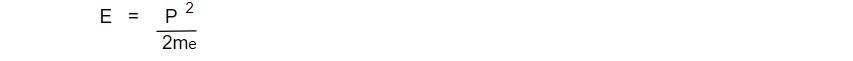The total energy of the electron gas is

E total = ΣE dN

Since the energy spectrum of non relativistic electron is continuous in the classical limit, we can summation by integration.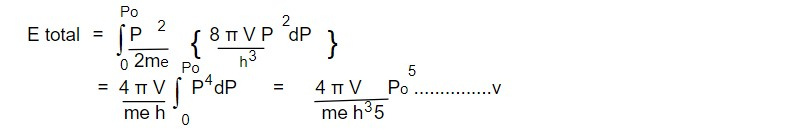Substituting the value of Po from eq iv, equation v takes the formWe know that the pressure exerted by electron gas is given by

Pgas = 2/3 E/V

For non relativistic electron gas,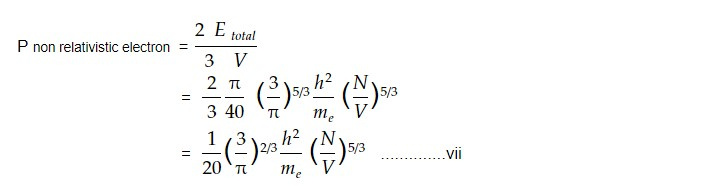This is our required expression. It is found that degeneracy pressure exerted by non relativistic electron is independent of temperature and depends only on the number density of degenerate gas.

The ratio (N/V) can be expressed in terms of mean molecular weight of gases in this way.,

ρ = m/ V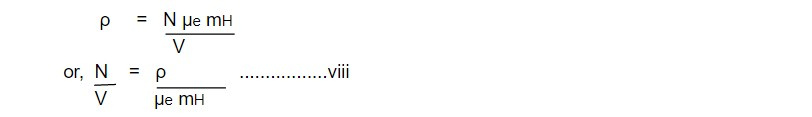Here µe is the mean molecular weight of free electrons. This can be calculated as,

µe = 1 / αX + βY + γZ

For hydrogen, α = 1/1 =1

β = 2/4 - atomic mass unit (2p + 2n)

γ = 1/2

Thus, µe = 2/ [X + (X + Y+ Z)]

= 2/ X + I [ as X+ Y+ Z = I]

The equation vii becomes,This is our required expression for the pressure exerted by non relativistic electrons in the star.

If the density is greater than 10^8 kgm³ in the stellar interior, the momenta of electrons are so large that their velocity approaches the speed of light. In this situation, the momentum exceeds the rest energy (i.e mec²) of electrons and the relativistic expression for the electron energy has to be used. In the extreme relativistic case

E total = ∫ E dN.....i

We know that the number of electrons in phase space having interval [ P, P+dP] is given by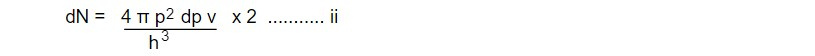Equation 1 becomeswhere Po is the limiting momentum acquired by the electrons due to temperature (as well as density fluctuation)To find the value of limiting momentum, one should integrate equation ii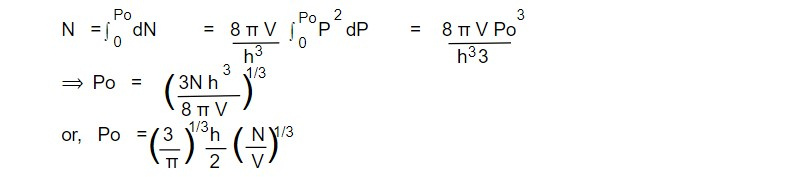Substituting the expression for Po from equation iv to equation iii we getIn the extreme relativistic limit, electron energy should be understand of photon energy (i.e mec² = hγ). The distribution of photon is in general isotropic and they are non interacting. Thus they satisfy the condition for degenerate matter. Here we use the expression for the radiation pressure.

The pressure exerted by photon gas is given by

P = 1 E total/ 3VThus, the pressure exerted by relativistic electron gas is given by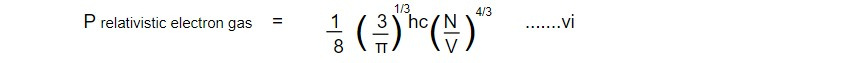Here the ratio (N/V) can be expressed in terms of mean molecular weight of free electron as,

N/ V = ρ / µe mH ...........vii

Thus equation 6 takes the from,This is our required expression for relativistic degenerate electron gas. It can be seen that this pressure is independent of temperature and depends upon ρ and chemical composition of the star (µe). Here, µe is given by

µe = 1/ αX + βY + γZ

β = 2/4 = 1/2

γ ~ 1/2 upto Fe

Thus,

µe = 2/ X+ X+Y+ Z

µe = 2/ X + 1 [ as X + Y+ Z = 1]

Then equation viii can be re written asThis is the generalized form of relativistic electron degeneracy pressure. It is noticeable that the fractional amount of hydrogen is different to calculated degeneracy pressure at a particular density.

E = p²/2m , v<<c

E = pc , v->c

In this way one can obtain the non relativistic and relativistic expression to the pressure. In intermediate cases, the expression for electron energy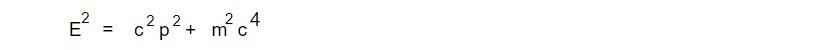has to be used.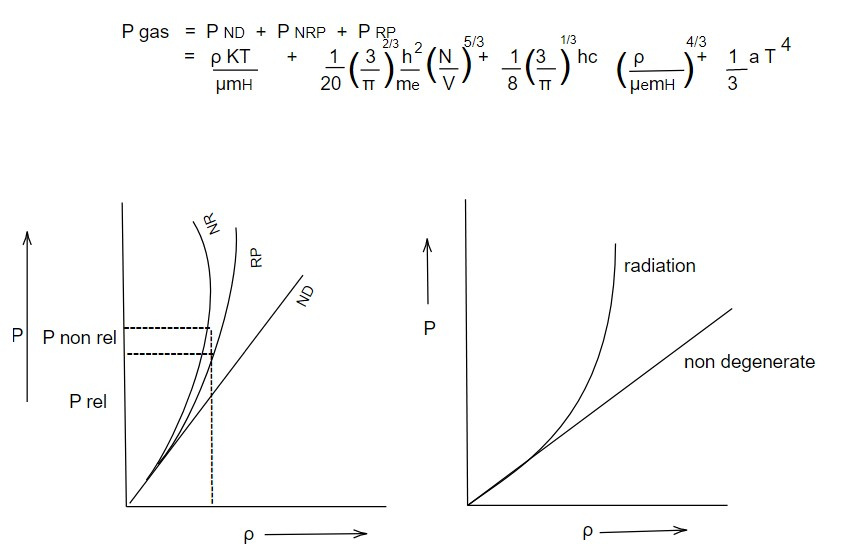This note is taken from Astrophysics, MSC Nepal.

This note is a part of the Physics Repository.